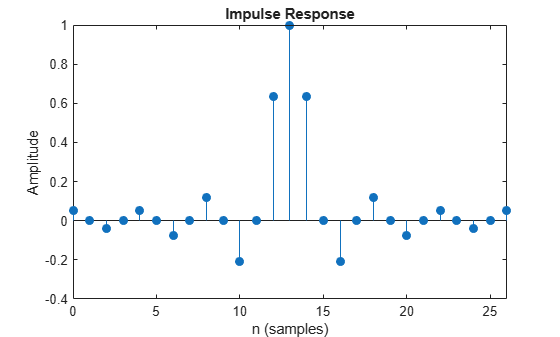# coeffs

Returns the filter System object coefficients in a structure

## Syntax

``s = coeffs(sysobj)``
``s = coeffs(sysobj,'Arithmetic',arithType)``

## Description

example

````s = coeffs(sysobj)` returns the coefficients of filter System object™, `sysobj`, in the structure `s`.```
````s = coeffs(sysobj,'Arithmetic',arithType)` returns filter coefficients for the filter System object `sysobj` with the arithmetic specified in `arithType`.```

## Examples

collapse all

```FIRHalfbandInterp = dsp.FIRHalfbandInterpolator('Specification',... 'Filter order and transition width','FilterOrder',26); C = coeffs(FIRHalfbandInterp); C.Numerator```
```ans = 1×27 0.0525 0 -0.0379 0 0.0537 0 -0.0771 0 0.1172 0 -0.2060 0 0.6345 1.0000 0.6345 0 -0.2060 0 0.1172 0 -0.0771 0 0.0537 0 -0.0379 0 0.0525 ```
```% Impulse response of the filter fvtool(FIRHalfbandInterp,'impulse')```## Input Arguments

collapse all

Arithmetic used in the filter analysis, specified as `'double'`, `'single'`, or `'Fixed'`. When the arithmetic input is not specified and the filter System object is unlocked, the analysis tool assumes a double-precision filter. When the arithmetic input is not specified and the System object is locked, the function performs the analysis based on the data type of the locked input.

The `'Fixed'` value applies to filter System objects with fixed-point properties only.

When the `'Arithmetic'` input argument is specified as `'Fixed'` and the filter object has the data type of the coefficients set to `'Same word length as input'`, the arithmetic analysis depends on whether the System object is unlocked or locked.

• unlocked –– The analysis object function cannot determine the coefficients data type. The function assumes that the coefficients data type is signed, has a 16-bit word length, and is auto scaled. The function performs fixed-point analysis based on this assumption.

• locked –– When the input data type is `'double'` or `'single'`, the analysis object function cannot determine the coefficients data type. The function assumes that the data type of the coefficients is signed, has a 16-bit word length, and is auto scaled. The function performs fixed-point analysis based on this assumption.

To check if the System object is locked or unlocked, use the `isLocked` function.

When the arithmetic input is specified as `'Fixed'` and the filter object has the data type of the coefficients set to a custom numeric type, the object function performs fixed-point analysis based on the custom numeric data type.

## Output Arguments

collapse all

Structure with a single field, `Numerator`, containing filter coefficients.

## Version History

Introduced in R2011a